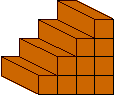Quandaries and Queries My son will be participating in the national math contest. He is a grade 4 student but will be writing the Fibonacci exam for grade 5. He has been given last years exam for practice. Parents have been ask to assist for this part. I was therfore hoping for a little "inside" help on several questions with answers & short explanations. This with a would be greatly appreciated.(from last years exam) With 3 weights, Mathusalem can weigh any object that has a weight which is smaller or equal to 13 kg, but that weighs a whole number of kilograms. He wants to weigh an object of 7 kilograms. To do so, he must place two of the weights on the left side of the scale, and the third one on the right side. Which one will he have to place on the right side a)4 b)3 c)6 d)2 e)5 Mathilda uses a rectangular prisms to build stairs. The cross section of each prism is a square which has a side of 1 dm. Mathilda has already put in place 4 steps of a staircase that must have a height of 10 dm. When finished, the staircase will have a volume of 550 dm3. What will the width of the staicase in dm be? a)10 b)55 c)11 d)1 e)100 The year 1991 was the last palindrome year of the second millennium. The year 2002 is the first palindrome year of the third millennium. Including 2002, how many palindrome years will there be, altogether, in the third millennium ? a)8 b)2 c)10 d)182 e) 9 Greatly appreciated, Mike Hi Mike, I think there must have been more information with these problems. Was there, for example a diagram? If Mathusalem can use 3 weights to weigh any object that is 13 kg or less then his weights must be 1 kg, 3 kg and 9 kg. To weigh his 7 kg object he must put it together with the 3 kg weight and balance them against the 9 kg and 1 kg weight on the other side. Since he put 2 weights on the left side he must have put the kg weight on the right side. I see a diagram like the one belowThese 4 steps have used 1+2+3+4=10 prisms. If the hieght is to be 10 steps it will take 1+2+3+...+10=55 prisms. Thus the area of the end is 55 square dm and hence, for a volume of 550 cubic dm the width of the staircase must be 10 dm. Palindromes in the third century have the form 2xx2 where x is a digit. Thus there are 10 palindromes since x can be 0,1,2,...,9. Cheers, Penny Go to Math Central